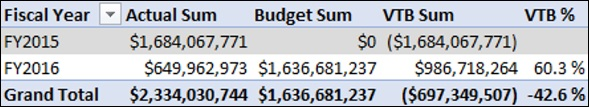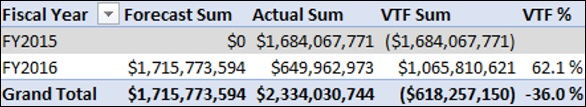# Variance Measures and Analysis

You can create variance measures such as variance to budget, variance to forecast, and forecast variance to budget. You can also analyze the finance data based on these measures.

### Creating Variance to Budget Sum Measure

Create Variance to Budget Sum measure (VTB Sum) as follows −

VTB Sum:=[Budget Sum]-[Actual Sum]

### Creating Variance to Budget Percentage Measure

Create Variance to Budget Percentage measure (VTB %) as follows −

VTB %:=IF([Budget Sum],[VTB Sum]/ABS([Budget Sum]),BLANK())

## Analyzing Data with Variance to Budget Measures

Create a Power PivotTable as follows −

• Add Fiscal Year from the Date table to Rows.
• Add the measures Actual Sum, Budget Sum, VTB Sum, VTB % from the Finance Data table to Values.### Creating Variance to Forecast Sum Measure

Create Variance to Forecast Sum (VTF Sum) measure as follows −

VTF Sum:=[Forecast Sum]-[Actual Sum]

### Creating Variance to Forecast Percentage Measure

Create Variance to Forecast Percentage measure (VTF %) as follows −

VTF %:=IF([Forecast Sum],[VTF Sum]/ABS([Forecast Sum]),BLANK())

## Analyzing Data with Variance to Forecast Measures

Create a Power PivotTable as follows −

• Add Fiscal Year from the Date table to Rows.
• Add the measures Actual Sum, Forecast Sum, VTF Sum, VTF % from the Finance Data table to Values.### Creating Forecast Variance to Budget Sum Measure

Create Forecast Variance to Budget Sum (Forecast VTB Sum) measure as follows −

Forecast VTB Sum:=[Budget Sum]-[Forecast Sum]

### Creating Forecast Variance to Budget Percentage Measure

Create Forecast Variance to Budget Percentage (Forecast VTB Percentage) measure as follows −

Forecast VTB %:=IF([Budget Sum],[Forecast VTB Sum]/ABS([Budget Sum]),BLANK())

## Analyzing Data with Forecast Variance to Budget Measures

Create a Power PivotTable as follows −

• Add Fiscal Year from the Date table to Rows.
• Add the measures Budget Sum, Forecast Sum, Forecast VTB Sum, Forecast VTB % from the Finance Data table to Values.